Instant Maths Help:

# Edexcel A Level

## Integration Basics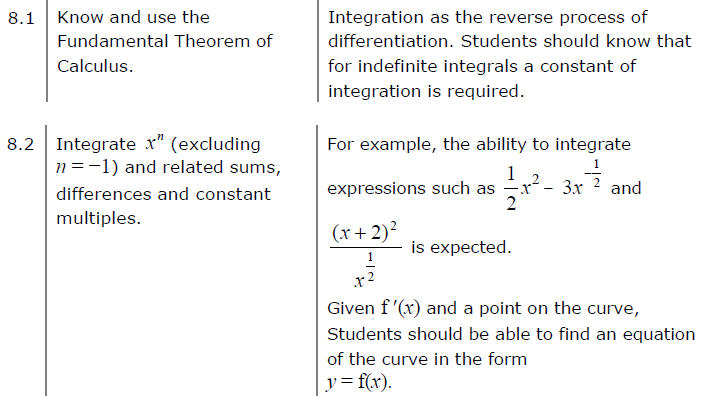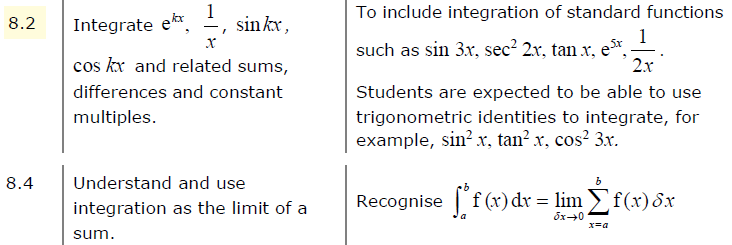##### Edexcel 9MA0/01 Jun 2023 A2 Exam Q. 1 :   4 marks in 4:48 min.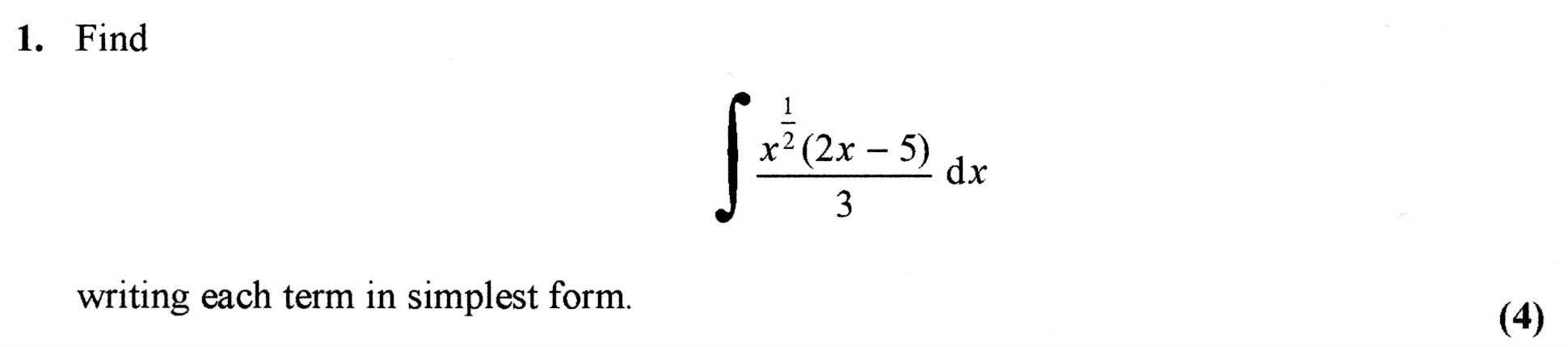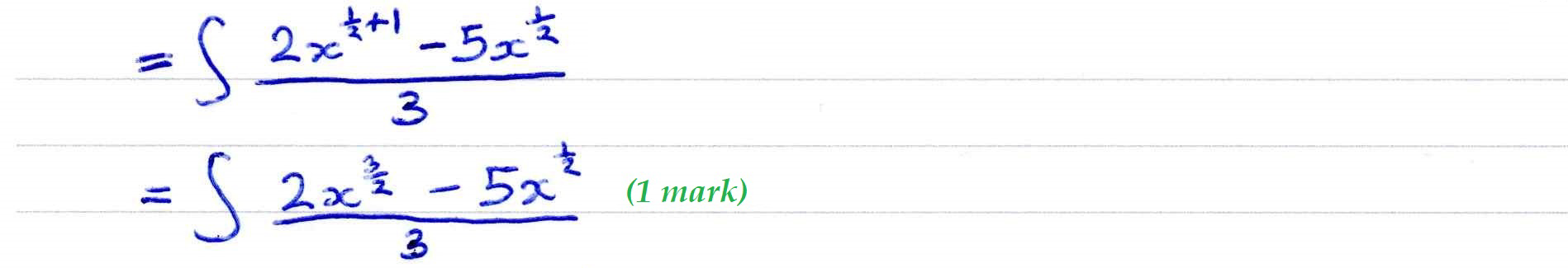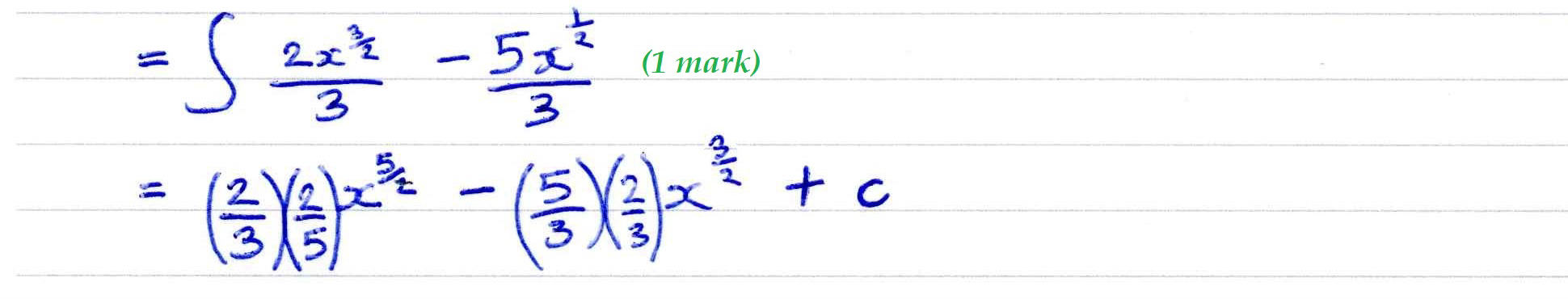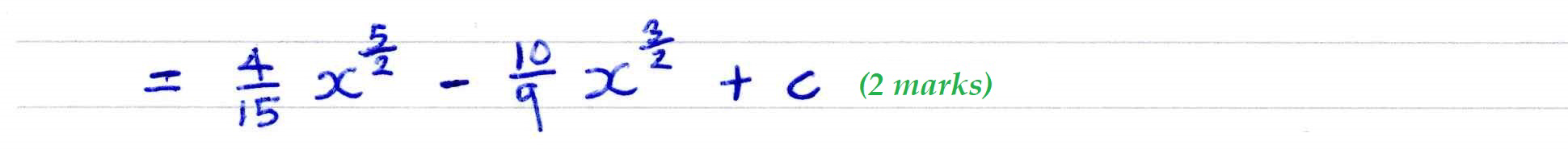##### Edexcel 8MA0/01 Jun 2023 AS Exam Q. 16 :   6 marks in 7:12 min.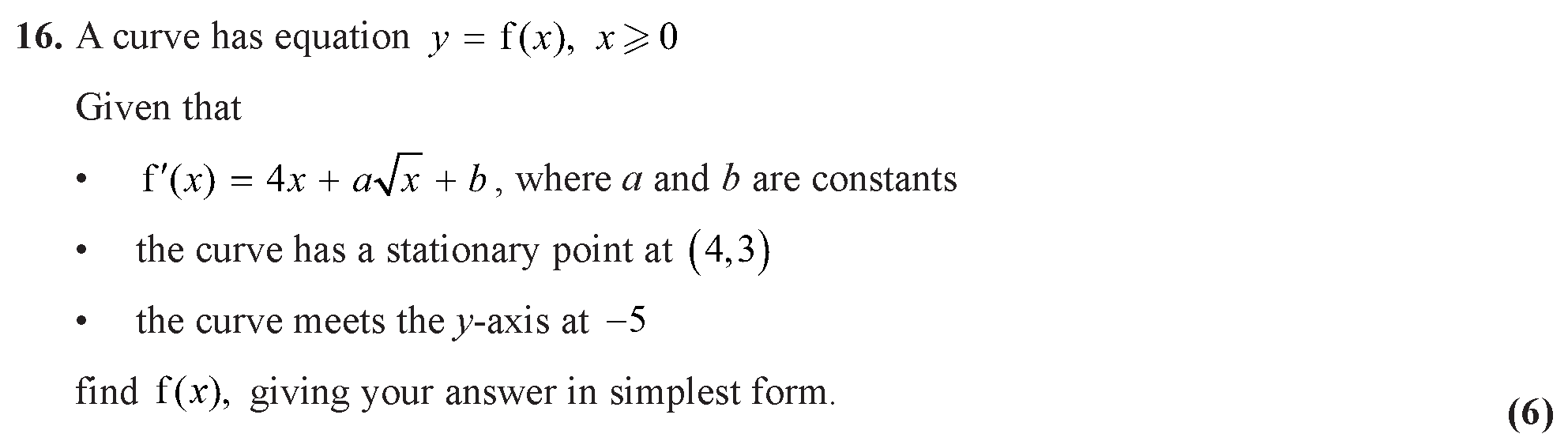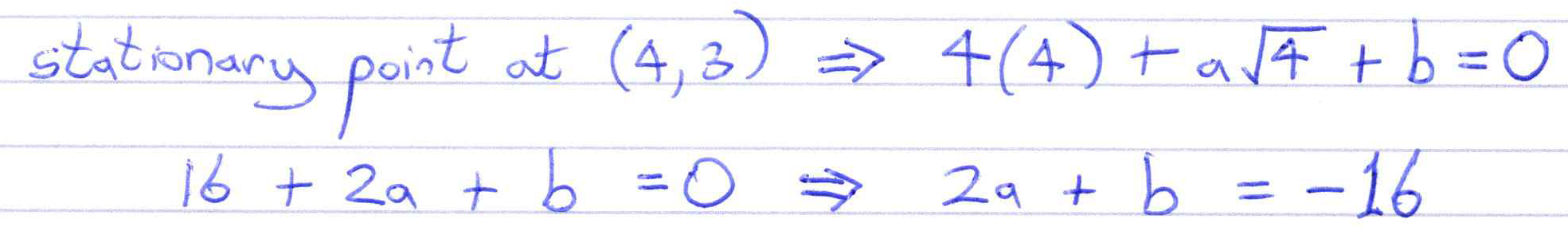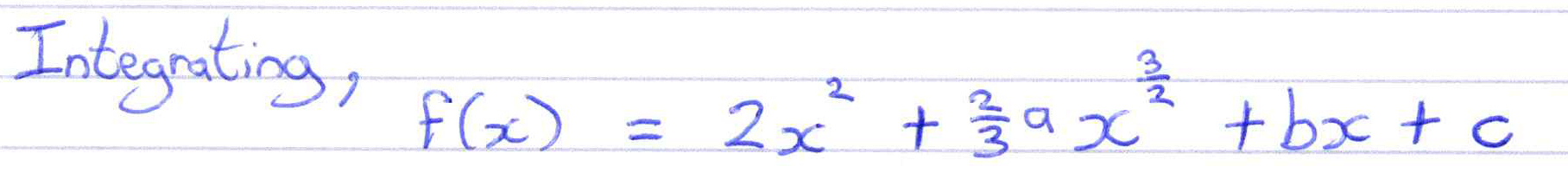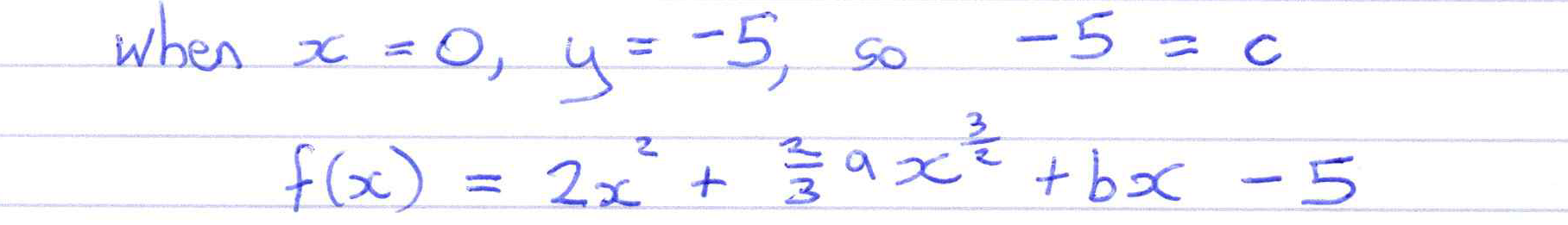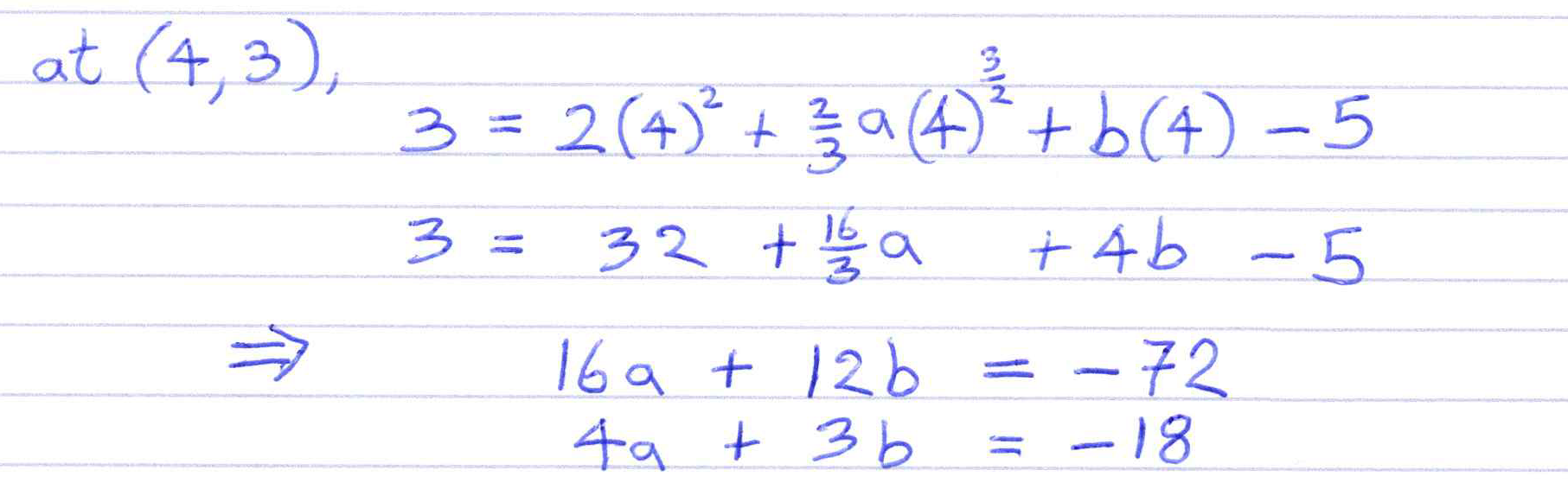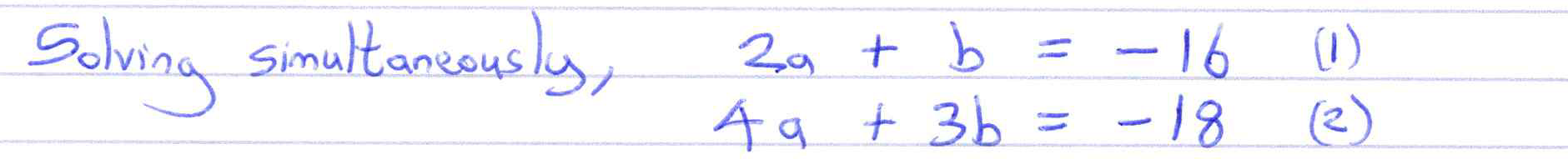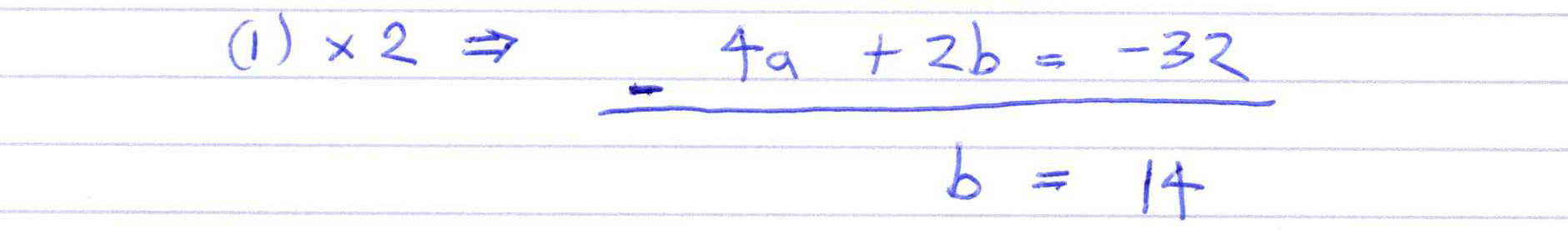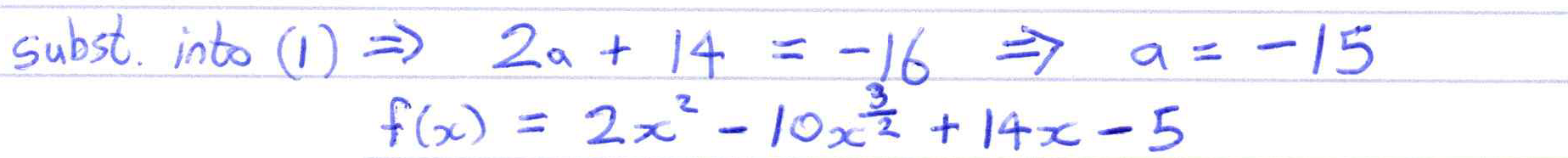##### Edexcel 9MA0/02 Jun 2023 A2 Exam Q. 5 :   5 marks in 6:00 min.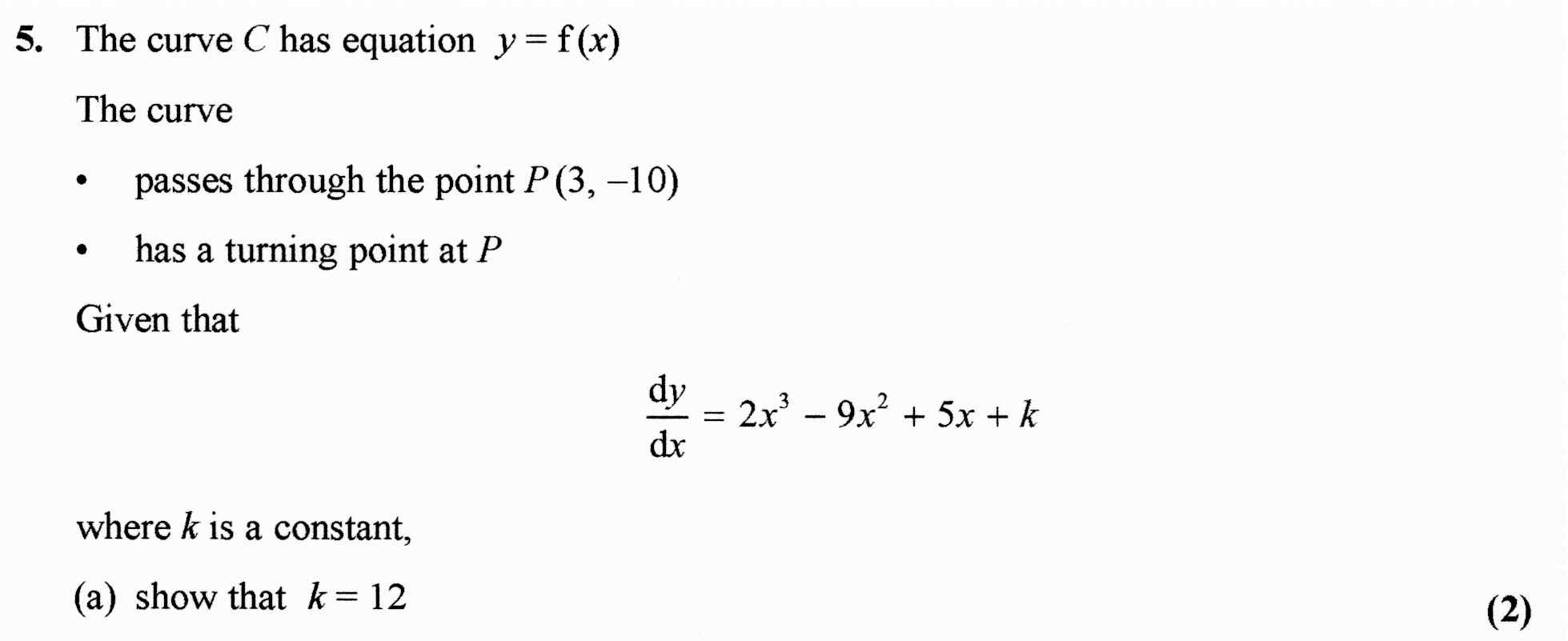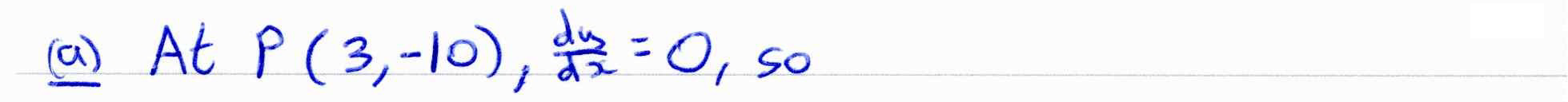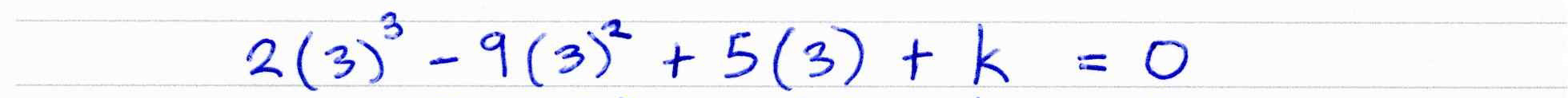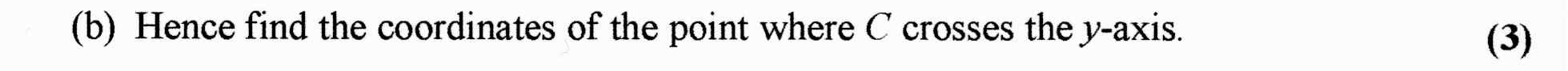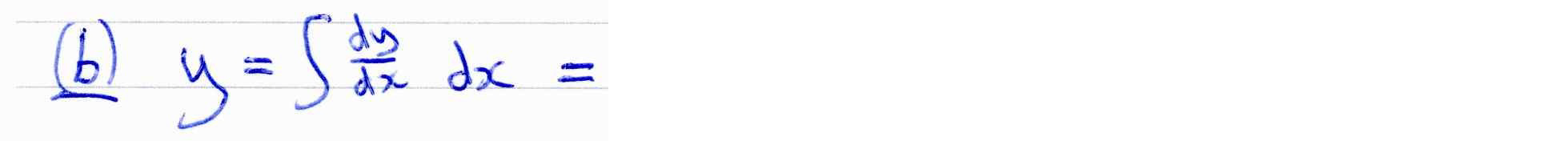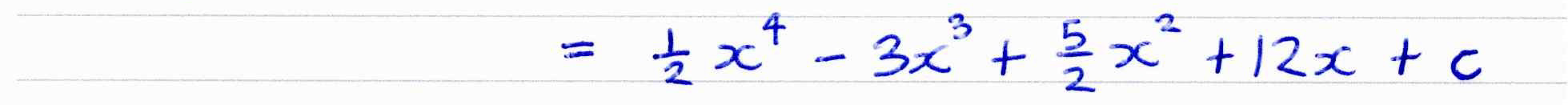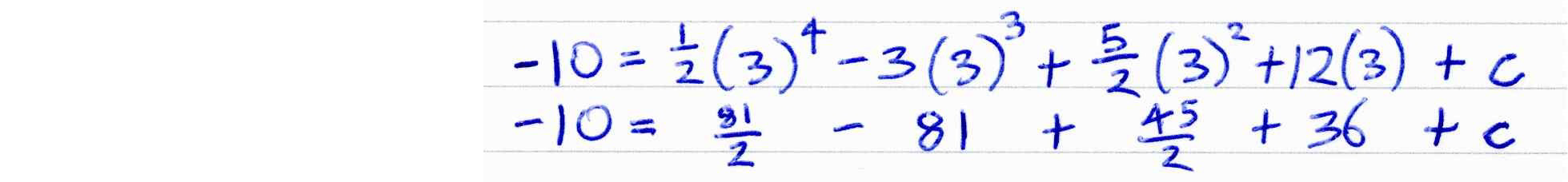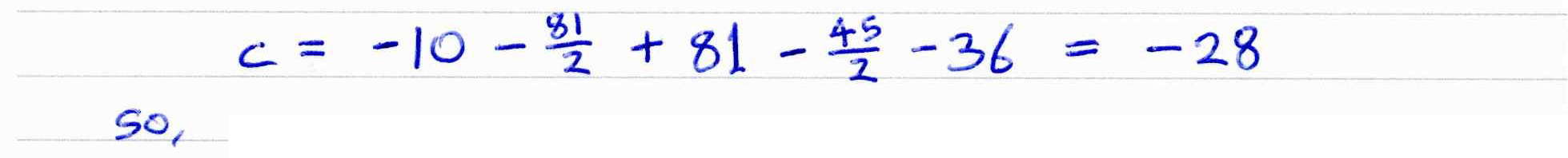##### Checking answers with numerical integration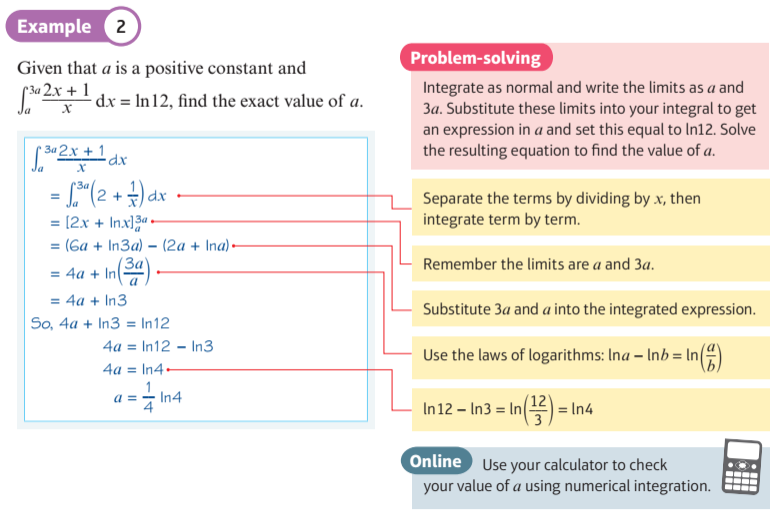##### Determining the constant of integration##### Investigate Integration of ${e^{ax}}$
This display allows you to visualise the integration of $\color{blue}{ { e ^ { \, a \, x } } }$ or $\color{blue}{ { e ^ { \, x } } }$ when a = 1.

The graph of the exponential function is shown in blue and the graph of the integral is in green.
The a-slider allows you to adjust the exponential function and the c-slider allows you to adjust the constant of integration in its integral.
The x-slider allows you to run along the curves to see correpondence at a single point.
##### Investigate Integration of ${ 1 \over { ax } }$
This display allows you to visualise the integration of $\color{blue}{ { 1 \over { a \, x } } }$ or $\color{blue}{ { 1 \over x } }$ when a = 1.

The graph of the reciprocal function is shown in blue and the graph of the integral is in green.
The a-slider allows you to adjust the exponential function and the c-slider allows you to adjust the constant of integration in its integral.
The x-slider allows you to run along the curves to see correpondence at a single point.
##### Investigate Trig Integration
This display allows you to visualise some trigonometric integrals.

The graph of the trigonometric function is shown in blue and the graph of the integral is in green.
The k-slider allows you to adjust the trigonometric function and the c-slider allows you to adjust the constant of integration in its integral.
The x-slider allows you to run along the curves to see correpondence at a single point.